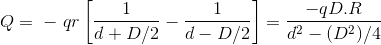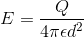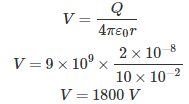# MCQs on Electromagnetic Fields

##### Page 9 of 20. Go to page 1 2 3 4 5 6 7 8 9 10 11 12 13 14 15 16 17 18 19 20
01․ Two identical circular coils, one closed and another is little split (not enclosed) are placed in an uniform magnetic field which decrease at a constant rate. If the plane to the coils are perpendicular to the field which of the following statements is true?
An emf is induced in both coils.
An emf is induced in split coil.
Equal joule heating occurs in both coils.
Joule heating does not occur in each of the coils.

Whenever a coil open or closed, cuts magnetic line of force, an emf is induced in it.

02․ A long wire composed of a smooth round conductor runs above and parallel to the ground (assumed to be a large conducting plane). A high voltage exits between the conductor and the ground. The maximum electric stress occur at
Midway between the conductor and ground.
The lower surface of the conductor.
The upper surface of the conductor.
The ground surface.

Since the distance from ground to lower surface is less than from ground to upper surface. So electric stress is maximum at lower surface.

03․ A loop is rotating about x-axis in a magnetic field, B = BO cos(ω + φ) ay. The voltage in the loop is
Due to both rotating and transformer action.
Due to rotating only.
Due to transformer only.
Zero.

Whenever a moving circuit is kept in a time varying magnetic field induced emf have two components: One for time variation of B and other for turn motion of circuit in B.

04․ A metal sphere with 1 m radius and a surface charge density of 10 coulombs/m2 is enclosed in a cube of 10 m side. The total outward electric displacement normal to the surface of the cube is
5 C.
10 C.
40π C.
None of above.

D = q × 4π r2.

05․ An electric dipole of moment P is placed in front of a ground sphere. The change induced on the surface of the sphere is (Assume radius of sphere = R and distance from dipole to centre of sphere = d)
Zero.
PR/d2.
PR 2/d3.
PR/d.

Charge induced on a spherical surface = - QR/D Where, Q = inducing point charge; D = distance of the point charge from the centre of sphere. Total charge induce in present case,Now for a dipole D is very small i.e. D << d Hence Q = PR/d2 where, P = qD, the dipole moment.

06․ What is the value of total electric flux coming out of a closed surface?
Zero.
Equal to volume change density.
Equal to total charge enclosed by the surface.
Equal to the surface charge density.

According to gauss theorem, the total electric flux through any closed surface surrounding charges is equal to the amount to charges enclosed by the surface.

07․ Electric field strength of charge
Decrease with distance.
Decrease with square distance.
Increase distance.
Increase square distance.

Electric field strength or electric field intensity.08․ The statement which is not correct from the following:
Gauss’s law states that total electric flux through any surface is equal to the total charge enclosed by surface.
For a uniform long charged line with l1 c/m the electric field intensity E is given as E = ρL/(π ε0.r).
An electric flux line is an imaginary path or a line drawn in such a way, that its direction at any point is the direction of electric field at that point.
Gauss’s static that divergence of D = ρu.

It is E = ρL/(2πε0.r).

09․ Two infinite parallel metal plates are charged with equal surface charge density of the same polarity. The electric field in the gap between the plates is
Zero.
Dependent on coordinates of field point.
Double of field produced by one plate.
The same as that produced by one plate.

For an infinite sheet of charge having surface charge density ρs, the electric field on either side is ρ/2ε and away from it assuming charge to be the positive). Therefore, in the region between the plates, the two fields are anti parallel and equal.

10․ An insulated metal sphere of 20 cm diameter is charged by rubbing with charges 2 × 10-8 C. Potential developed will be
0 V.
200 V.
900 V.
1800 V.

r = d/2.<<<7891011>>>